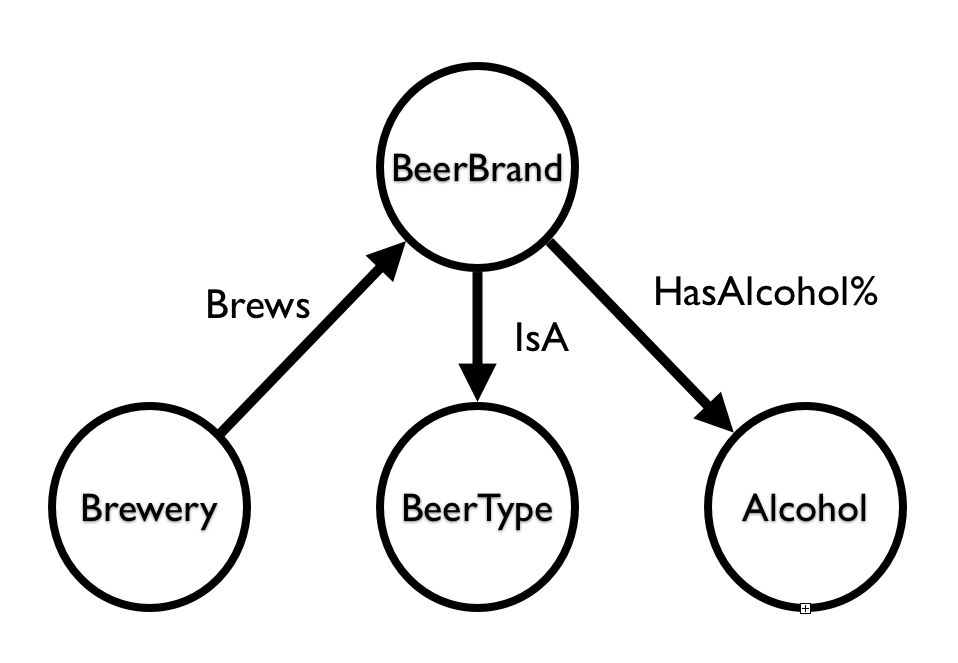# Belgian Beer Graph - straight from Wikipedia into a GraphGist

For the past couple of years, I have been preaching the www.neo4j.com[Neo4j] gospel in many different places, meetups, conferences, and what have you. For the most part, I have been using a very specific demo that has been super well-received: The Belgian Beer Graph. It started out as a learning experience for me personally back in the day when Neo4j did not have any proper data import functionality - and I had to load the graph by jumping through all kinds of hoops.

That of course has changed so much in the mean while. We have wonderful data loading capabilities these days, and in this GraphGist I would like to show you a lovely end-to-end data loading example based on

Sounds easy enough? Let’s explore.

``````=query({IMPORTHTML("https://nl.wikipedia.org/wiki/Lijst_van_Belgische_bieren";"table";2);
IMPORTHTML("https://nl.wikipedia.org/wiki/Lijst_van_Belgische_bieren";"table";3);
IMPORTHTML("https://nl.wikipedia.org/wiki/Lijst_van_Belgische_bieren";"table";4);
IMPORTHTML("https://nl.wikipedia.org/wiki/Lijst_van_Belgische_bieren";"table";5);
IMPORTHTML("https://nl.wikipedia.org/wiki/Lijst_van_Belgische_bieren";"table";6);
IMPORTHTML("https://nl.wikipedia.org/wiki/Lijst_van_Belgische_bieren";"table";7);
IMPORTHTML("https://nl.wikipedia.org/wiki/Lijst_van_Belgische_bieren";"table";8);
IMPORTHTML("https://nl.wikipedia.org/wiki/Lijst_van_Belgische_bieren";"table";9);
IMPORTHTML("https://nl.wikipedia.org/wiki/Lijst_van_Belgische_bieren";"table";10);
IMPORTHTML("https://nl.wikipedia.org/wiki/Lijst_van_Belgische_bieren";"table";11);
IMPORTHTML("https://nl.wikipedia.org/wiki/Lijst_van_Belgische_bieren";"table";12);
IMPORTHTML("https://nl.wikipedia.org/wiki/Lijst_van_Belgische_bieren";"table";13);
IMPORTHTML("https://nl.wikipedia.org/wiki/Lijst_van_Belgische_bieren";"table";14);
IMPORTHTML("https://nl.wikipedia.org/wiki/Lijst_van_Belgische_bieren";"table";15);
IMPORTHTML("https://nl.wikipedia.org/wiki/Lijst_van_Belgische_bieren";"table";16);
IMPORTHTML("https://nl.wikipedia.org/wiki/Lijst_van_Belgische_bieren";"table";17);
IMPORTHTML("https://nl.wikipedia.org/wiki/Lijst_van_Belgische_bieren";"table";18);
IMPORTHTML("https://nl.wikipedia.org/wiki/Lijst_van_Belgische_bieren";"table";19);
IMPORTHTML("https://nl.wikipedia.org/wiki/Lijst_van_Belgische_bieren";"table";20);
IMPORTHTML("https://nl.wikipedia.org/wiki/Lijst_van_Belgische_bieren";"table";21);
IMPORTHTML("https://nl.wikipedia.org/wiki/Lijst_van_Belgische_bieren";"table";22);
IMPORTHTML("https://nl.wikipedia.org/wiki/Lijst_van_Belgische_bieren";"table";23);
IMPORTHTML("https://nl.wikipedia.org/wiki/Lijst_van_Belgische_bieren";"table";24);
IMPORTHTML("https://nl.wikipedia.org/wiki/Lijst_van_Belgische_bieren";"table";25);
IMPORTHTML("https://nl.wikipedia.org/wiki/Lijst_van_Belgische_bieren";"table";26);
IMPORTHTML("https://nl.wikipedia.org/wiki/Lijst_van_Belgische_bieren";"table";27);
IMPORTHTML("https://nl.wikipedia.org/wiki/Lijst_van_Belgische_bieren";"table";28)};
"select * where Col1 <> 'Merk'";0)``````

Super easy. It will dynamically update the data in the sheet - so effectively we can now scrape Wikipedia and immediately make it available for loading into Neo4j from a CSV export. All we now need to do is make the sheet available to the public so that LOAD CSV can use the URL to download the dataset, and make sure the AlcoholPercentages are loaded correctly as numerical values.

Since there are quite a few Belgian Beers (yey!) we need to load a larger dataset into this GraphGist - so this may take a bit of time. The model that we would be using to import the data into is very simple:``````create index on :BeerBrand(name);
create index on :Brewery(name);
create index on :BeerType(name);
create index on :AlcoholPercentage(value);

with csv as beercsv
where beercsv.BeerType is not null
merge (b:BeerType {name: beercsv.BeerType})
with beercsv
where beercsv.BeerBrand is not null
merge (b:BeerBrand {name: beercsv.BeerBrand})
with beercsv
where beercsv.Brewery is not null
merge (b:Brewery {name: beercsv.Brewery})
with beercsv
where beercsv.AlcoholPercentage is not null
merge (b:AlcoholPercentage {value: tofloat(replace(replace(beercsv.AlcoholPercentage,'%',''),',','.'))})
with beercsv
match (ap:AlcoholPercentage {value: tofloat(replace(replace(beercsv.AlcoholPercentage,'%',''),',','.'))}),
(br:Brewery {name: beercsv.Brewery}),
(bb:BeerBrand {name: beercsv.BeerBrand}),
(bt:BeerType {name: beercsv.BeerType})
create (bb)-[:HAS_ALCOHOLPERCENTAGE]->(ap),
(bb)-[:IS_A]->(bt),
(bb)<-[:BREWS]-(br);``````

The dataset if a bit too big for visualising in one Graph, but we can of course take a sample with 10 BeerBrands and their relationships:

``````match (b:BeerBrand)
with b
limit 10
match (b)--(n)
return b,n;``````

Let’s look at the sample:

And then we can do our typical Beer queries

``````MATCH (orval:BeerBrand {name:"Orval"})
return orval;``````

And again we look at the result:

Or one of my favourites: finding the paths between "Duvel" and "Orval" beers

``````MATCH (duvel:BeerBrand {name:"Duvel"}), (orval:BeerBrand {name:"Orval"}),
p = AllshortestPaths( (duvel)-[*]-(orval) )
RETURN p;``````

It’s still a great example of a typical pathfinding operation that is yielding interesting "beer recommendations" - something I can always appreciate :) …​